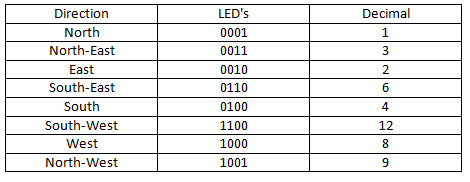## Audio

[Audio][slideshow]

Description.
Here is the circuit diagram of a very simple and accurate electronic compass using the 1490 digital compass from Dinsmore. The 1490 digital compass is a solid state Hall Effect device which is sensitive to detect the Earth’s magnetic field. The sensor is internally damped to simulate the performance of an ordinary compass. The circuit shown here is the simplest one that can be built around this sensor.

The sensor has 12 pins at the bottom which are arranged in to four sets of three. All pins marked 1 are connected to the positive (+5V). All pins marked 2 are tied together and connected to the ground. The pins marked 3 are the output pins. Each output pin is equivalent to the open collected of an NPN transistor and can sink up to 20mA. An LED is connected between the positive (+5V) and each of the output pin. The 1K resistor will limit the LED current to 5mA.The direction can be identified by interpreting the combination of LEDs glowing. A typical 5V regulator using IC 7805 is used to produce 5V DC from the 9V battery for powering the circuit.

For the calibration of the compass circuit, you need an ordinary magnetic compass. Determine the North using the standard compass. Now, rotate the compass so that only one LED glows. Take this LED as the North. The sensor does not have a fixed North and you can actually use any output as the North. If the circuit is wired exactly as show in circuit diagram, the other three outputs will also fall in the proper sequence. In my circuit the LED D4 was the North. Rotating the digital compass in the clock wise direction will make the LEDs to glow in the following sequence where 1=ON and 0=OFF.Circuit diagram.Notes.

• Assemble the circuit a good quality PCB.
• Using sub miniature LEDs will increase the battery life.
• The circuit can be powered from a 9V PP3 battery.
• Ferrous materials close to the sensor will affect its performance.

[Lights][grids]